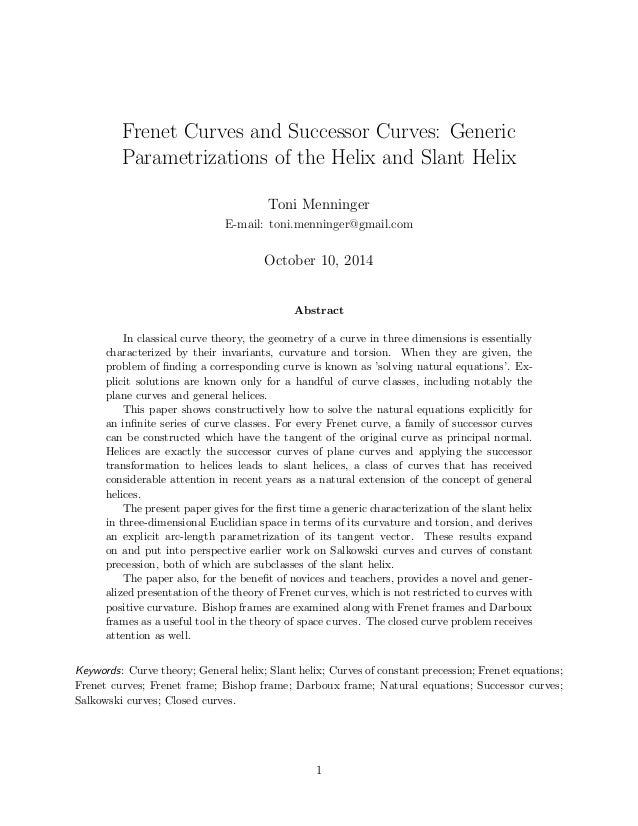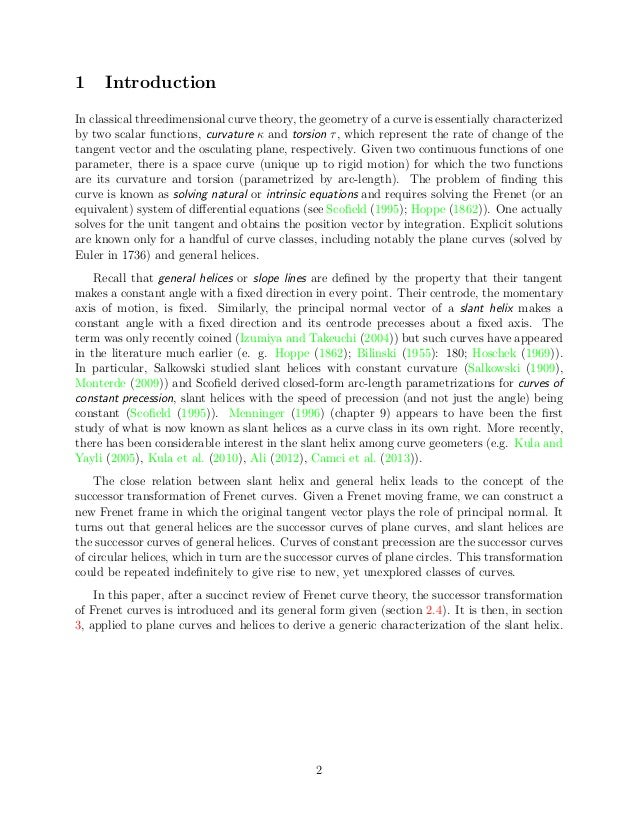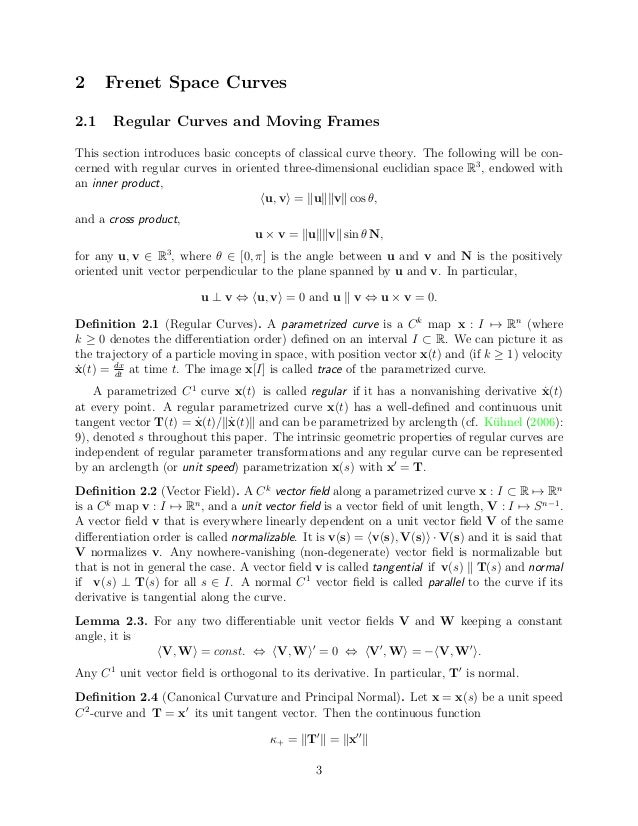Successfully reported this slideshow.Upcoming SlideShare
×

of0

Share

# Frenet Curves and Successor Curves: Generic Parametrizations of the Helix and Slant Helix

In classical curve theory, the geometry of a curve in three dimensions is essentially characterized by their invariants, curvature and torsion. When they are given, the problem of finding a corresponding curve is known as 'solving natural equations'. Explicit solutions are known only for a handful of curve classes, including notably the plane curves and general helices.

This paper shows constructively how to solve the natural equations explicitly for an infinite series of curve classes. For every Frenet curve, a family of successor curves can be constructed which have the tangent of the original curve as principal normal. Helices are exactly the successor curves of plane curves and applying the successor transformation to helices leads to slant helices, a class of curves that has received considerable attention in recent years as a natural extension of the concept of general helices.

The present paper gives for the first time a generic characterization of the slant helix in three-dimensional Euclidian space in terms of its curvature and torsion, and derives an explicit arc-length parametrization of its tangent vector. These results expand on and put into perspective earlier work on Salkowski curves and curves of constant precession, both of which are subclasses of the slant helix.

The paper also, for the benefit of novices and teachers, provides a novel and generalized presentation of the theory of Frenet curves, which is not restricted to curves with positive curvature. Bishop frames are examined along with Frenet frames and Darboux frames as a useful tool in the theory of space curves. The closed curve problem receives attention as well.

See all

### Related Audiobooks

#### Free with a 30 day trial from Scribd

See all
• Be the first to like this

### Frenet Curves and Successor Curves: Generic Parametrizations of the Helix and Slant Helix

1. 1. Frenet Curves and Successor Curves: Generic Parametrizations of the Helix and Slant Helix Toni Menninger E-mail: toni.menninger@gmail.com October 10, 2014 Abstract In classical curve theory, the geometry of a curve in three dimensions is essentially characterized by their invariants, curvature and torsion. When they are given, the problem of
2. 2. nding a corresponding curve is known as 'solving natural equations'. Ex- plicit solutions are known only for a handful of curve classes, including notably the plane curves and general helices. This paper shows constructively how to solve the natural equations explicitly for an in
3. 3. nite series of curve classes. For every Frenet curve, a family of successor curves can be constructed which have the tangent of the original curve as principal normal. Helices are exactly the successor curves of plane curves and applying the successor transformation to helices leads to slant helices, a class of curves that has received considerable attention in recent years as a natural extension of the concept of general helices. The present paper gives for the
4. 4. rst time a generic characterization of the slant helix in three-dimensional Euclidian space in terms of its curvature and torsion, and derives an explicit arc-length parametrization of its tangent vector. These results expand on and put into perspective earlier work on Salkowski curves and curves of constant precession, both of which are subclasses of the slant helix. The paper also, for the bene
5. 5. t of novices and teachers, provides a novel and gener- alized presentation of the theory of Frenet curves, which is not restricted to curves with positive curvature. Bishop frames are examined along with Frenet frames and Darboux frames as a useful tool in the theory of space curves. The closed curve problem receives attention as well. Keywords: Curve theory; General helix; Slant helix; Curves of constant precession; Frenet equations; Frenet curves; Frenet frame; Bishop frame; Darboux frame; Natural equations; Successor curves; Salkowski curves; Closed curves. 1
6. 6. 1 Introduction In classical threedimensional curve theory, the geometry of a curve is essentially characterized by two scalar functions, curvature and torsion , which represent the rate of change of the tangent vector and the osculating plane, respectively. Given two continuous functions of one parameter, there is a space curve (unique up to rigid motion) for which the two functions are its curvature and torsion (parametrized by arc-length). The problem of
7. 7. nding this curve is known as solving natural or intrinsic equations and requires solving the Frenet (or an equivalent) system of dierential equations (see Sco
8. 8. eld (1995); Hoppe (1862)). One actually solves for the unit tangent and obtains the position vector by integration. Explicit solutions are known only for a handful of curve classes, including notably the plane curves (solved by Euler in 1736) and general helices. Recall that general helices or slope lines are de
9. 9. ned by the property that their tangent makes a constant angle with a
10. 10. xed direction in every point. Their centrode, the momentary axis of motion, is
11. 11. xed. Similarly, the principal normal vector of a slant helix makes a constant angle with a
12. 12. xed direction and its centrode precesses about a
13. 13. xed axis. The term was only recently coined (Izumiya and Takeuchi (2004)) but such curves have appeared in the literature much earlier (e. g. Hoppe (1862); Bilinski (1955): 180; Hoschek (1969)). In particular, Salkowski studied slant helices with constant curvature (Salkowski (1909), Monterde (2009)) and Sco
14. 14. eld derived closed-form arc-length parametrizations for curves of constant precession, slant helices with the speed of precession (and not just the angle) being constant (Sco
15. 15. eld (1995)). Menninger (1996) (chapter 9) appears to have been the
16. 16. rst study of what is now known as slant helices as a curve class in its own right. More recently, there has been considerable interest in the slant helix among curve geometers (e.g. Kula and Yayli (2005), Kula et al. (2010), Ali (2012), Camci et al. (2013)). The close relation between slant helix and general helix leads to the concept of the successor transformation of Frenet curves. Given a Frenet moving frame, we can construct a new Frenet frame in which the original tangent vector plays the role of principal normal. It turns out that general helices are the successor curves of plane curves, and slant helices are the successor curves of general helices. Curves of constant precession are the successor curves of circular helices, which in turn are the successor curves of plane circles. This transformation could be repeated inde
17. 17. nitely to give rise to new, yet unexplored classes of curves. In this paper, after a succinct review of Frenet curve theory, the successor transformation of Frenet curves is introduced and its general form given (section 2.4). It is then, in section 3, applied to plane curves and helices to derive a generic characterization of the slant helix. 2
18. 18. 2 Frenet Space Curves 2.1 Regular Curves and Moving Frames This section introduces basic concepts of classical curve theory. The following will be con-cerned with regular curves in oriented three-dimensional euclidian space R3, endowed with an inner product, hu; vi = kukkvk cos ; and a cross product, u v = kukkvk sin N; for any u; v 2 R3, where 2 [0; ] is the angle between u and v and N is the positively oriented unit vector perpendicular to the plane spanned by u and v. In particular, u ? v , hu; vi = 0 and u k v , u v = 0: De
19. 19. nition 2.1 (Regular Curves). A parametrized curve is a Ck map x : I7! Rn (where k 0 denotes the dierentiation order) de
20. 20. ned on an interval I R. We can picture it as the trajectory of a particle moving in space, with position vector x(t) and (if k 1) velocity x_ (t) = dx dt at time t. The image x[I] is called trace of the parametrized curve. A parametrized C1 curve x(t) is called regular if it has a nonvanishing derivative x_ (t) at every point. A regular parametrized curve x(t) has a well-de
21. 21. ned and continuous unit tangent vector T(t) = x_ (t)=kx_ (t)k and can be parametrized by arclength (cf. Kuhnel (2006): 9), denoted s throughout this paper. The intrinsic geometric properties of regular curves are independent of regular parameter transformations and any regular curve can be represented by an arclength (or unit speed) parametrization x(s) with x0 = T. De
22. 22. nition 2.2 (Vector Field). A Ck vector
23. 23. eld along a parametrized curve x : I R7! Rn is a Ck map v : I7! Rn, and a unit vector
24. 24. eld is a vector
25. 25. eld of unit length, V : I7! Sn

Total views

950

On Slideshare

0

From embeds

0

Number of embeds

27

6

Shares

0